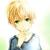## 一个Java面试题，求解释原因

为什么80%的码农都做不了架构师？->>>public class Test {

public static void main(String args[]) {

StringBuffer a = new StringBuffer("A");

StringBuffer b = new StringBuffer("B");

Operator(a, b);

System.out.println(a + " " + b);

}

public static void Operator(StringBuffer x, StringBuffer y) {

x.append(y);

y = x;

}

}

public class Test {

public static void main(String args[]) {

StringBuffer a = new StringBuffer("A");

StringBuffer b = new StringBuffer("B");

Operator(a, b);

System.out.println(a + " " + b);

}

public static void Operator(StringBuffer x, StringBuffer y) {

x.append(y);

y = x;

}

}

1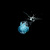1.首先你在main方法中创建了两个StringBuffer对象,它们的引用分别指向的值是"A"和"B";

2.调用operator()方法时,因为你这里传递的是引用,会发生形参引用拷贝的过程!所以这里又新创建了两个对象,注意是又新创建了两个对象!但是这两个新创建的对象的引用也还是指向你在main方法中创建的两个StringBuffer对象.意思就是你形参中的x和main中的a这两个现在的指针地址是一样的,同理形参中y与main中的b这两个指针地址也是一样的.

3.在operator方法中,x.append(y);这个过程实际是改变x指针指向的值,注意是指向的值!值与指针是两个不同的概念!值和指针不能混淆!因为x指针指向的值发生了改变,所以main中的a的指针指向的值也发生了改变!对了,这里提一下,指针有内存地址,值也有内存地址,他们的内存地址是不一样的,你可以通过c语言的%p格式化输出;

4.operator方法中,y = x;这里步骤实际上是改变形参y的指针地址!但是并没有改变main中b的指针地址!因此此时形参的y打印输出的结果会是"AB",但是y的作用域只有在operator方法中.而main中b的指针地址仍然指向"B",所以格式化输出"B";0

0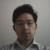Operator(a, b); 传到 Operator 方法中的是 a 和 b 的地址的复制品，在 Operator 方法中 y = x; 修改的是 b 的地址复制品 y，并不会把 b 的地址设置成 a 的地址。
0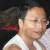0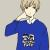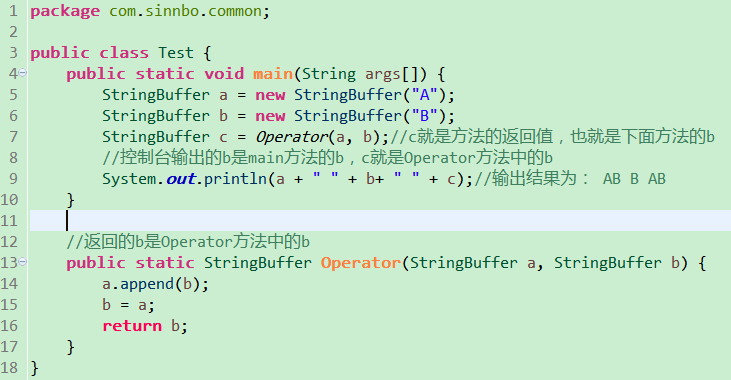0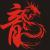```public static void operator(StringBuffer x,StringBuffer y){
// a 的拷贝变为了AB，a 的拷贝(x)和a 指向同一个内存地址，所以 a 变为了AB
x.append(y);
//  y 指向了 a 的拷贝的地址，也就是 a 的地址，那么y 将不再影响 b
y=x;
}```

0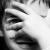```StringBuffer a = new StringBuffer("A");
StringBuffer b = new StringBuffer("B");
//这两句话声明两个引用对象 a和b,在内存中分别指向"A","B"两个对象在内存中的地址

x.append(y);
//这句话就是把x指向对象的值修改为"AB"(这样说是因为StringBuffer是可变的),修改后内存地址不变,所以x和a还是指向原来的对象,只是对应的值变成了"AB"
y=x;
//这句话会把此时x存储的内存地址赋值给y,此时y就和x就指向同一个对象,同理y就和a指向同一个对象.此时b中存储的内存地址一直没有变化.

a="AB", b="B"

public class TestStackHeap {

public static void main(String[] args) {
StringBuffer a = new StringBuffer("A");
StringBuffer b = new StringBuffer("B");
StringBuffer m = swap(a, b);
System.out.println("main: a=" + a + ", b=" + b);
System.out.println("a == m is " + (a == m));
}

public static StringBuffer swap(StringBuffer x, StringBuffer y) {
x.append(y);
y = x;
System.out.println("swap: x=" + x + ", y=" + y);
return y;
}

}

swap: x=AB, y=AB
main: a=AB, b=B
a == m is true
==================================================================
public class TestStackHeap {

public static void main(String[] args) {
String a = new String("A");
String b = new String("B");
String m = swap(a, b);
System.out.println("main: a=" + a + ", b=" + b);
System.out.println("a == m is " + (a == m));
}

public static String swap(String x, String y) {
x = x + y;
y = x;
System.out.println("swap: x=" + x + ", y=" + y);
return y;
}

}
swap: x=AB, y=AB
main: a=A, b=B
a == m is false

```

0